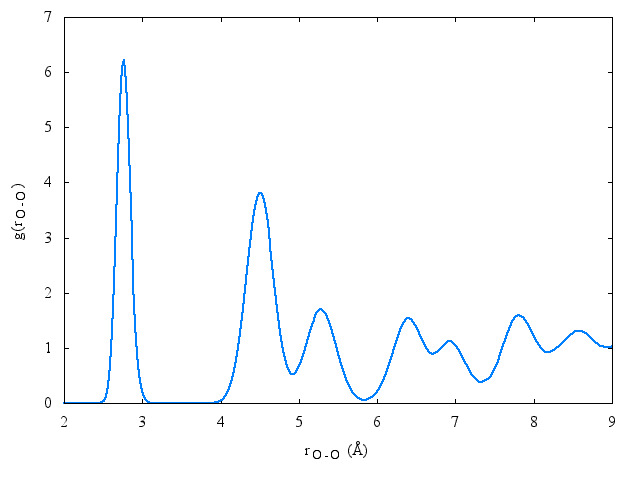# Ice Ih

Ice Ih, also known as hexagonal ice, is a proton disordered ice phase having the space group P63/mmc. Ice Ih has the following lattice parameters at 250 K: a=4.51842 Å,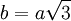$b=a\sqrt3$, and c=7.35556 Å with four molecules per unit cell (in Table 3 of ). The proton ordered form of ice Ih is known as ice XI, which (in principle) forms when ice Ih is cooled to below 72K (it is usually doped with KOH to aid the transition).

## Melting point

The following is a collection of melting points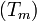$(T_m)$ for the ice Ih-water transition (in ascending order):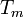$T_m$ Pressure Water model / technique Reference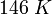$146~K$ 1 bar TIP3P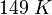$149~K$ 1 bar COS/G3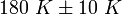$180~K \pm 10~K$ 1 bar POL3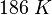$186~K$ 1 bar SWM4-DP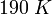$190~K$ 1 bar SPC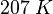$207~K$ 1 bar BKd1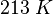$213~K$ 1 bar BKd2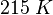$215~K$ 1 bar COS/G2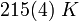$215(4)~K$ 1 bar SPC/E / free energy calculation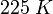$225~K$ 1 bar TTM3-F (quantum)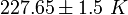$227.65 \pm 1.5~K$ 1 bar TTM2.1-F (quantum)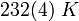$232(4)~K$ 1 bar TIP4P / free energy calculation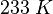$233~K$ 1 bar BKd3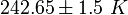$242.65 \pm 1.5~K$ 1 bar TTM2.1-F (classical)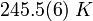$245.5(6)~K$ 1 bar TIP4P/Ew / free energy calculation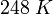$248~K$ 1 bar TTM3-F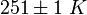$251 \pm 1~K$ 1 bar q-TIP4P/F / direct coexistence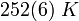$252(6)~K$ 1 bar TIP4P/2005 / free energy calculation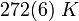$272(6)~K$ 1 bar TIP4P/Ice / free energy calculation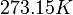$273.15 K$ 1 bar experimental value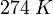$274~K$ 1 bar TIP5P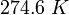$274.6~K$ 1 bar mW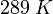$289~K$ 1 bar NvdE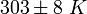$303\pm 8~K$ 1 bar TIP4P/FQ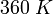$360~K$ 1 bar BLYP-D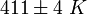$411 \pm 4~K$ 10,000 bar BLYP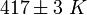$417\pm 3~K$ 2500 bar Perdew-Burke-Ernzerhof functional 

Isotopes:$T_m$ (D20) Pressure Water model / technique Reference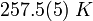$257.5(5)~K$ 1 bar q-TIP4P/F / free energy calculation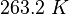$263.2~K$ 1 bar TIP4PQ_D2O / free energy calculation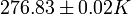$276.83 \pm 0.02 K$ 1 bar experimental value$T_m$ (T20) Pressure Water model / technique Reference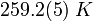$259.2(5)~K$ 1 bar q-TIP4P/F / free energy calculation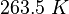$263.5~K$ 1 bar TIP4PQ_T2O / free energy calculation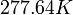$277.64 K$ 0.6629 kPa experimental value 

It is worth pointing out that the calculations presented in the work of Ramírez and Herrero  used the melting point of the q-TIP4P/F model as its "reference state". It is perhaps more fruitful to examine the relative changes upon isotopic substitution: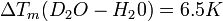$\Delta T_m (D_2O - H_2 0) = 6.5 K$ (experimental value: 3.68 K) and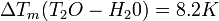$\Delta T_m (T_2O - H_2 0) = 8.2 K$ (experimental value: 4.49 K).

## Radial distribution function

The following is a plot of the oxygen-oxygen radial distribution function for the TIP4PQ/2005 model at 77K